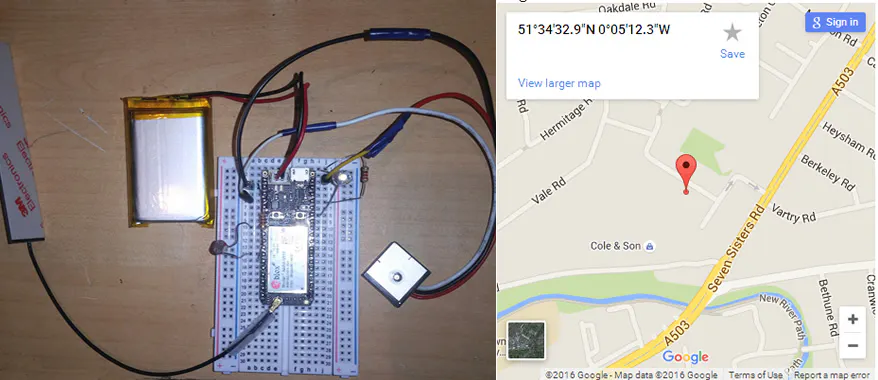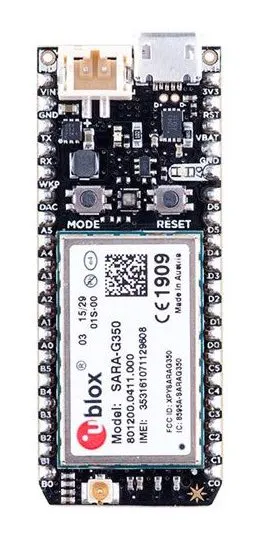Published

# Cheap and Simple Electron GPS

BeginnerShowcase (no instructions)1 hour5,508## Things used in this project

### Hardware componentsParticle Electron
×1
×1

## Code

### Electron Code

C/C++
```// GPSTest

String inWord;
char inByte;
String data;

void setup() {
// cloud variable
Particle.variable("STU", data);
// GPS Serial
Serial1.begin(9600);
}

void loop() {
// read serial data from GPS unit
while (Serial1.available() > 0) {

if (inByte == '\n') {
// END OF LINE

// check is data we want
// you can change this to get any data line values
if (inWord.startsWith("\$GPRMC")) {
// put data string in variable
data = inWord;
// clear the inword variable
inWord = "";
} else {
// clear the inword variable as not the data we want
inWord = "";
}

} else {
// build data string
inWord += inByte;
}

} // end if serial
} // end loop
```

### Webpage code

PHP
Publish on a web server and use any browser to view the location of your Electron
```<?PHP
# particle-map.php
// GPS UNIT
// https://cdn.sparkfun.com/datasheets/GPS/GP-20U7.pdf

\$deviceID = "YOUR_PARTICLE_DEVIC_ID";
\$access_token = "YOUR_PARTICLE_ACCESS_TOKEN";

\$url = "https://api.particle.io/v1/devices/\$deviceID/";
\$formed_url ='?access_token='.\$access_token;
\$variable_name = "STU";

"GET /v1/devices/".\$variable_name.\$formed_url." HTTP/1.1",
"Host: api.particle.io");

// setup and make HTTP GET REQUEST
\$ch = curl_init();
curl_setopt(\$ch, CURLOPT_URL,\$url.\$variable_name.\$formed_url);
curl_setopt(\$ch, CURLOPT_RETURNTRANSFER, true); // return output
\$retrievedhtml = curl_exec (\$ch);
curl_close(\$ch);
\$json = json_decode(\$retrievedhtml,true);

// see if there was an error connecting with electron
if (\$json['error'] != "") {
echo ("ERROR = " . \$json['error'] . "<br>");
} else {
// read the data into a variable
\$DATA = \$json['result'];
// output to screen
echo ("<b>result: </b>" . \$DATA . "<br>");
// split data into array  is comma delimited
\$pieces = explode(",", \$DATA);
// A = valid, V = not valid
\$status = \$pieces;
if (\$status == "V") {
echo ("Data not valid<br>Can the GPS unit see the sky?");
} else {
// put data in variables
\$LAT = \$pieces;
\$LON = \$pieces;
\$EW = \$pieces;
// Convert LAT
\$deg = substr(\$LAT, 0, 2);
\$min = substr(\$LAT, 2, 8);
\$sec = '';
\$resultLAT = \$deg+(((\$min*60)+(\$sec))/3600);
print ("Latitude " . \$resultLAT . "<br>");

// Convert Longitude
\$deg = substr(\$LON, 0, 3);
\$min = substr(\$LON, 3, 8);
\$sec='';
\$resultLON = \$deg+(((\$min*60)+(\$sec))/3600);

// Is it East or West
if (\$EW == "W") {
\$resultLON = \$resultLON * -1;
}

print ("Longitude " . \$resultLON . "<br>");
}
}
?>

<iframe
width="400"
height="400"
frameborder="0" style="border:0"